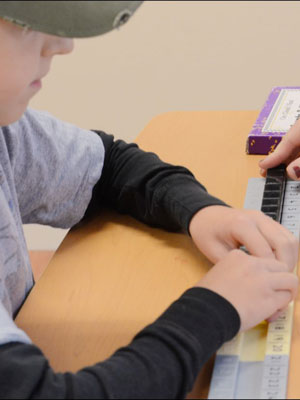# Math Programs and Math Tutoring

### The On Cloud Nine ® Math Program

The On Cloud Nine ® math program provides development of Numeral Imagery and Concept Imagery in order to improve mathematical concepts and computation by developing a dual-coding base of imagery and language, which improves the ability to think and reason with numbers.

Improved math skills will be developed through the integration of language with manipulatives and visual imagery. This will aid in the understanding of arithmetic concepts and accuracy in computation. Course Outline:

• Imaging Numerals
• Visualizing and Verbalizing the Number Line
• Addition and Subtraction Family Facts
• Word Problems
• Place Value
• Jumping with the Tens for Mental Adding and Subtracting
• Multiplication and Division
• Fractions and Decimals## Math Evaluations

Test of Mathematical Abilities (TOMA-3)

*Vocabulary

*Computation

*Math in everyday life

*Word problems

Math evaluation takes up to one hour. The consultation is scheduled on a different day to go over the results of the initial evaluation.

When your child or loved one needs math tutoring or help from a math tutor we hope you will consider calling our learning center in Wasilla, AK.